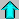# Astronomy 12 - Spring 1999 (S.T. Myers)

## The Speed of Light

Reference: See "Spacetime Physics" by E.F. Taylor and J.A. Wheeler
(W.H. Freeman, LoC 65-13566).

I was asked by a curious student why the speed of light is a constant according to relativity (and all experimental evidence). At the time I was not able to think of a satisfying explanation. Recently, however, I came across something in a book on spacetime physics that I found to be insightful.

The speed of light c is just the conversion factor between time and space! Just like there is a conversion factor between meters and miles, there is one between seconds and meters, and you can see that it is

c = 2.998 x 108 m/s.

We are used to the idea of distances being constant no matter what coordinate system is used - the distance between Philadelphia and New York is the same number of meters whether we use the normal North-South/East-West map grid, or one centered on Philadelphia for example. This is demonstrated by Pythagoras' theorem:

r2 = x2 + y2 + z2

You get the same r if you interchange the x,y,z or even rotate the axes (as long as you keep x,y,z all perpendicular to each other) and of course use the same units like meters.

In relativity, space and time are parts of the same thing, so a similar rule holds. However, time and space are different. In this case, the interval

R2 = (c t)2 - x2 - y2 - z2 = (ct)2 - r2

is an invariant! Note however that the time interval enters in with the opposite sign as the space interval - this is why time is different than space! Note for R^2 to be positive and to make sense, r < ct or the physical distance between two points must be less than the light-travel time between them. For R=0, r=ct is the distance traveled by light - light travels on "null" paths (called null geodesics) where the space-time interval R^2=0! The speed of light is a constant because light must follow these paths where the interval R^2 = 0.

When I discussed flat spacetime geometries in the lectures on cosmology, I stated that the geometry was Euclidean. This is not strictly true, if it were Euclidean, then Pythagoras' theorem would hold (in all 4 dimensions), and

R2 = (ct)2 + x2 + y2 + z2 = (ct)2 + r2

Note the difference in sign of how the space enters relative to the time. The geometry of spacetime is called Lorentzian - only the space part is Euclidean.

Note: if you know about complex numbers you will notice that the space part enters as if it were imaginary

R2 = (ct)2 + (ix)2 + (iy)2 + (iz)2 = (ct)2 + (ir)2

where i^2 = -1 as usual. This turns out to be the essence of the fabric (or metric) of spacetime geometry - that space enters in with the imaginary factor i relative to time.

If you've read Stephen Hawking's "A Brief History of Time", you might remember he discussed just this point. His postulate is that before the time of quantum gravity (in the Planck Era) the geometry WAS Euclidean, but became Lorentizan after the Planck transition. Kinda cool, eh?Return to Lecture 19 ---Astro12 Index ---Astro12 Home

smyers@nrao.edu Steven T. Myers